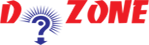﻿ Python Program for Fibonacci Sequence## Python Program to Print the Fibonacci Sequence

Here you will learn how to print the Fibonacci Sequence by using while loop. A Fibonacci sequence is the sequence of integers from 0, 1, 1, 2, 3, 5, 8......

The initial two terms are 0 and 1 and rest of the numbers are obtained simply by adding the preceeding two terms.

``````
Python Code
# Program to display the Fibonacci sequence up to n-th term where n is provided by the user

# change this value for a different result
nterms = 10

# uncomment to take input from the user
#nterms = int(input("How many terms? "))

# first two terms
n1 = 0
n2 = 1
count = 0

# check if the number of terms is valid
if nterms <= 0:
print("Please enter a positive integer")
elif nterms == 1:
print("Fibonacci sequence upto",nterms,":")
print(n1)
else:
print("Fibonacci sequence upto",nterms,":")
while count < nterms:
print(n1,end=' , ')
nth = n1 + n2
# update values
n1 = n2
n2 = nth
count += 1
Output
Fibonacci sequence upto 10 :
0, 1, 1, 2, 3, 5, 8, 13, 21, 34,.....
```
```

So learners see the tutorial and try it by changing the values of num in the program.

## Other Python Programs

PythonTraining, 258-FF , Katewa Nagar, New Sanganer Rd, Jaipur, 302019